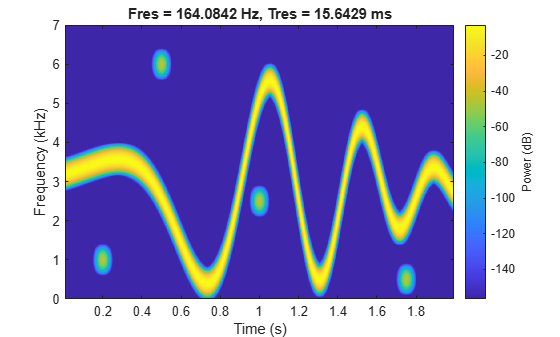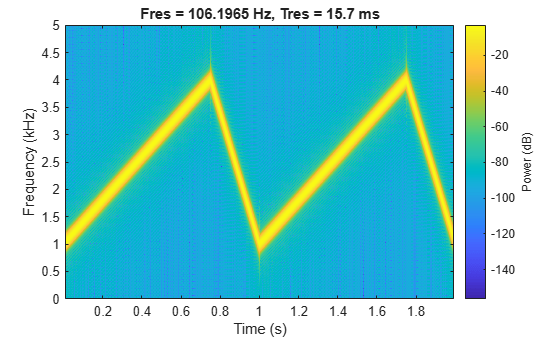# vco

Voltage-controlled oscillator

## Syntax

``y = vco(x,fc,fs)``
``y = vco(x,[Fmin Fmax],fs)``

## Description

example

````y = vco(x,fc,fs)` creates a signal that oscillates at a frequency determined by the real input vector or matrix `x` with sampling frequency `fs`. If `x` is a matrix, `vco` produces a matrix whose columns oscillate according to the columns of `x`.```

example

````y = vco(x,[Fmin Fmax],fs)` scales the frequency modulation range so that ±1 values of `x` yield oscillations of `Fmin` Hz and `Fmax` Hz, respectively.```

## Examples

collapse all

Generate two seconds of a signal composed of a voltage-controlled oscillator (vco) and four Gaussian atoms. The instantaneous frequency is modulated by a chirp function. The sample rate is 14 kHz.

```fs = 14000; t = (0:1/fs:2)'; gaussFun = @(A,x,mu,f) exp(-(x-mu).^2/(2*0.01^2)).*sin(2*pi*f.*x)*A'; s = gaussFun([1 1 1 1],t,[0.2 0.5 1 1.75],[10 60 25 5]*100)/10; x = vco(chirp(t+.1,0,t(end),3).*exp(-2*(t-1).^2),0.2*fs,fs); s = s/10+x;```

Plot the spectrogram of the generated signal. Specify 90% overlap and moderate spectral leakage.

`pspectrum(s,fs,'spectrogram','OverlapPercent',90,'Leakage',0.5)`Generate two seconds of a signal sampled at 10 kHz whose instantaneous frequency is a triangle function of time.

```fs = 10000; t = 0:1/fs:2; x = vco(sawtooth(2*pi*t,0.75),[0.1 0.4]*fs,fs);```

Plot the spectrogram of the generated signal. Specify the leakage as `0.80` and the overlap between adjoining segments as 95%.

`pspectrum(x,fs,'spectrogram','Leakage',0.80,'OverlapPercent',95)`## Input Arguments

collapse all

Input data, specified as a real vector or real matrix. `x` ranges from `–1` to `1`, where `x` = `–1` corresponds to 0 frequency output, `x` = `0` corresponds to `fc`, and `x` = `1` corresponds to `2*fc`.

Carrier or reference frequency used to modulate the input signal, specified as a real positive scalar.

Frequency modulation range limits, specified as a real vector. For best results, `Fmin` and `Fmax` should be in the range 0 to `fs/2`.

Note

vco performs FM modulation using the `modulate` function.

Sample rate, specified as a positive scalar. The sample rate is the number of samples per unit time. If the unit of time is seconds, then the sample rate has units of Hz.

## Output Arguments

collapse all

Oscillating output signal, returned as a real vector or real matrix. `y` is the same size as `x` and has amplitude equal to `1`.

## Version History

Introduced before R2006a